-=+=- -=+=- -=+=- -=+=- -=+=- -=+=- -=+=- -=+=- -=+=- -=+=- -=+=- -=+=- -=+=- -=+=- -=+=- -=+=- -=+=- -=+=- -=+=- -=+=- -=+=- -=+=- -=+=- -=+=- -=+=- -=+=- -=+=- -=+=- -=+=- -=+=- (c) WidthPadding Industries 1987 0|198|0 -=+=- -=+=- -=+=- -=+=- -=+=- -=+=- -=+=- -=+=- -=+=- -=+=- -=+=- -=+=- -=+=- -=+=- -=+=- -=+=- -=+=- -=+=- -=+=- -=+=- -=+=- -=+=- -=+=- -=+=- -=+=- -=+=- -=+=- -=+=- -=+=- -=+=- SoCoder -> Showcase Home -> Graphic Demos Retro styled Demos, showing off your graphical and musical abilities. Older --> Petri Dish Andy_A , BlitzPlus (should work in B3D as well) WindowsBiomorph Fractals A littlle known class of fractals Andy_A Blitz WindowsGrey Lined Backgrounds Grey Texture Generator Pakz Monkey IFS Viewer 3 IFS fractal viewer with benefits Andy_A Blitz Windows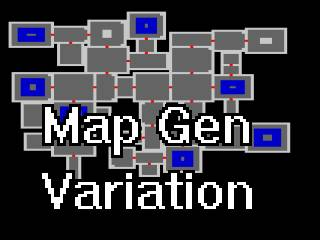Map Generator variation Rooms and doors and things in rooms Pakz Monkey Cross Platform Curlicue Fractals A different kind of fractal Andy_A Blitz Windows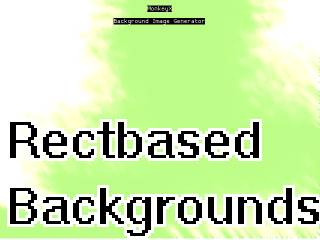Grey backgrounds Several graphical filters in action Pakz Monkey Cross PlatformMojo2 html5 animation A nice looking background animation effect Pakz Monkey Cross PlatformJulia Set Visualizer Render 2D + pseudo-3d Julia Sets Andy_A WindowsSVG Tech Demo 1 Quick tech demo showing an SVG image of a star with a gradient being deformed. The star has 80 points for a detailed deformation. HoboBen , Cobra WindowsCircles some circles, end of. JL235 Ruby Cross Platformtoon rendering create a cartoon 3d looking realtime Blitz SpinningCubesB3D Spins cubes around and then blows them apart! zebeste Blitz WindowsDutch Colourz GFX Demo shockwave QBasic WindowsDefacto2 Intro Liquorice intro! shockwave 2D Terrain Generator Old skool 2.5D Terrain Generator in B+ GUI Andy_A Blitz Windows Laser Show Just a simple laser show zebeste Basic Windows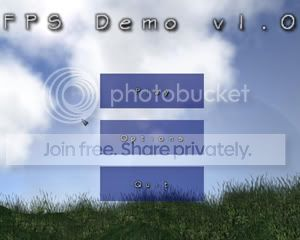{WIP} FPS Alpha Demo Alpha demo for a coming FPS game. Toaster Blitz WindowsBump Mapping Just a short short demo of blitz3D and bump mapping.. Toaster Blitz Windows 3D rotating starfield classic demo effect, looks pretty cool BdR Blitz WindowsVectorballs Demo another classic demo effect BdR Blitz Windows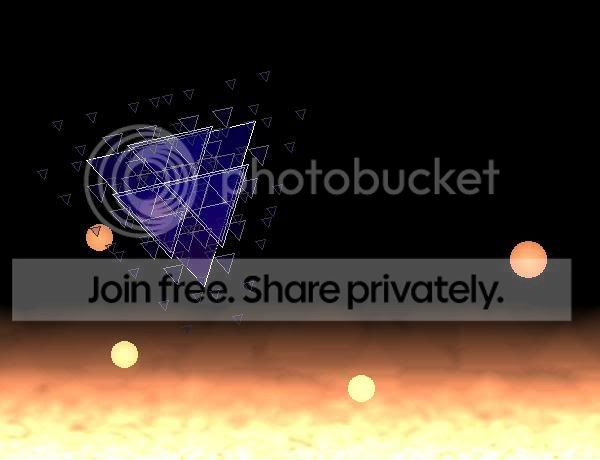Lavapit Demo mike_g Blitz ff8 mimic trying to mimic ff8 technique realtime BlitzAxial Product V4 Shows the magical world between square and circular (includes soundtrack) Teasy Blitz Windows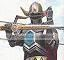here is Wolfie :) power mousey Blitz Windows Older -->